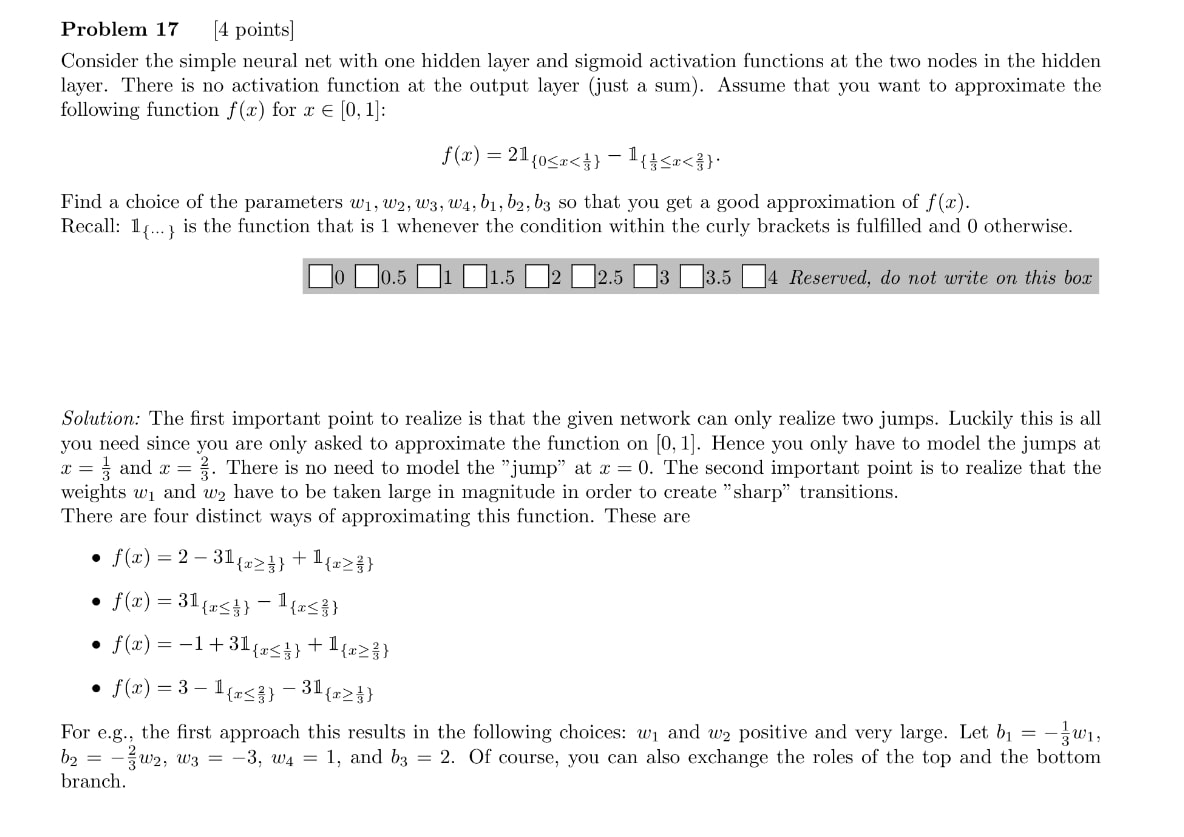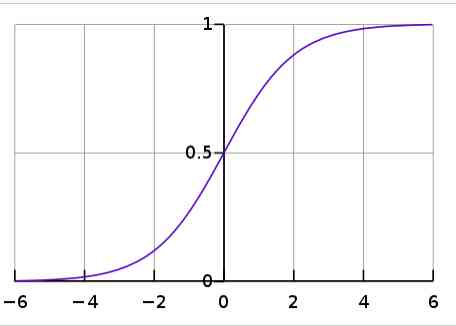### 2018 Exam Problem 17

Hi, I am massively confused by the following despite staring at it for a while. I simply do not understand how we move from the first function stated to the 4 approximation variants. I would be very grateful if someone could explain their thought process of how they would tackle it, as I do not understand the solutionary. Thank you so very muchlook at the slides over sigmoid functions with one layer which can approximate every functions moreless, I believe it will be then more clear for you. (lectures 8 I think)

Thanks, actually not very difficult at all. It wasn't even about checking the slides but just taking a break and coming back to it. Thanks for the help

Hi! The slides help a lot but I'm still struggling to adapt it to problem 17. In our case we have a rectangle of height 2 from x=0 to x=1/3 and another of height -1 from x=1/3 to x=2/3. As a function of sigmoids, this would give us ϕ(wx)-2ϕ(w(x-1/3))+ϕ(w(x-2/3)) and then I kind of just don't know where to go from there. I understand the given solution but I'm struggling to visualise how to get from the given functions to a sigmoid approximation.

Also I don't get why b1 and b2 are negative in the solution for the 1st approximation.

The key here is realising we want to approximate f(x) on [0,1], otherwise the problem is impossible to solve.

Draw f(x) and draw any of the solutions, you'll see they coïncide on that interval.

As seen in class, a 2 node 1 hidden layer NN can approximate a rectangle by combining two step functions (two sigmoids with high w)

Hi, this I understand I by drawing f(x) I see that the given solutions work for our problem. I also get that "a 2 node 1 hidden layer NN can approximate a rectangle by combining two step functions (two sigmoids with high w)". I think the confusion for me here is
1) Here we have two "rectangles" for f(x) so for me this requires more than two sigmoids
2) I'm struggling to visualise the given solutions in sigmoid approximation. If you have an example of how to express for example the 1st solution as two sigmoid functions that would help a lot.

Also in terms of methodology, is the key part here to rewrite f(x) as a function of upper bounds (i.e. 1/3 > x) rather than double bounds (i.e. 2/3>x>1/3)?

If you agree you can approximate a step with a sigmoid with large w, just consider a step is a sigmoid.. then by construction it's easy to see with the given solutions.

1) would be true if we cared about what happened outside the interval
2) Indeed the key is to upper bound only because that's how you define a step function.

Aah ok I see it now, thanks a lot! One last thing, I'm still confused as to why b1 and b2 are negative? I found the same values but positive as we are on [0,1].

You need to write the output of the neural network as a function of x by including the weights w1, ..., w4 and bias terms b1, b2, b3 just as for any neural net, and then identify the weights and bias variables with the function containing the phi(..) terms. For example if you take phi(w(x-2/3)) and if it should be identified with phi(w1x + b1) it would be rewritten phi(wx - 2/3w) that would lead you to have w1 = w (unknown for w1 and w2, but fixed for w3 and w4, you will see in the calculation) and b1 =-2/3w

Edit: Here we are assuming that the sigmoid function goes from 0 to 1id

Page 1 of 1